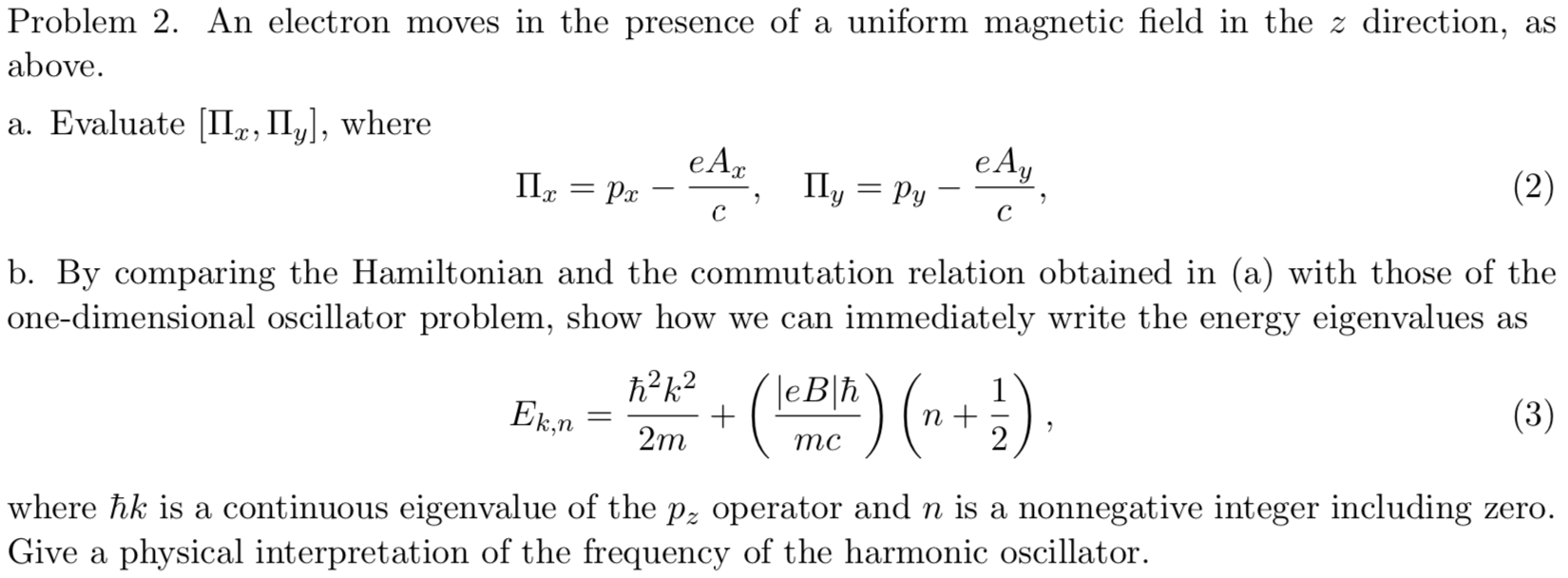# Problem 2. An electron moves in the presence of a uniform magnetic field in the z direction, as above. a. Evaluate II, II, where e А, eAy Py Ily (2) Ра с с b. By comparing the Hamiltonian and the commutation relation obtained in (a) with those of the one-dimensional oscillator problem, show how we can immediately write the energy eigenvalues as h2k2 leBh 1 Ek.n (3) 2m тс where hk is a continuous eigenvalue of the p2 operator and n is a nonnegative integer including zero. Give a physical interpretation of the frequency of the harmonic oscillator

Question

It's a quantum mechanics problem.help_outlineImage TranscriptioncloseProblem 2. An electron moves in the presence of a uniform magnetic field in the z direction, as above. a. Evaluate II, II, where e А, eAy Py Ily (2) Ра с с b. By comparing the Hamiltonian and the commutation relation obtained in (a) with those of the one-dimensional oscillator problem, show how we can immediately write the energy eigenvalues as h2k2 leBh 1 Ek.n (3) 2m тс where hk is a continuous eigenvalue of the p2 operator and n is a nonnegative integer including zero. Give a physical interpretation of the frequency of the harmonic oscillator fullscreen